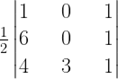NCERT Solutions for Maths Class 12 Exercise 4.3

# NCERT Solutions for Maths Class 12 Exercise 4.3

## NCERT Solutions for Maths Class 12 Exercise 4.3

### Maths Class 12 Ex 4.3 Question 1.

Find the area of the triangle with vertices at the point given in each of the following:
(i) (1, 0), (6, 0), (4, 3)
(ii) (2, 7), (1, 1), (10, 8)
(iii) (–2, –3), (3, 2), (–1, –8)

Solution:
(i) Area of a triangle =1/2 [1(0 3) + 1(18 0)]
1/2 [1 × (3) + 1 × 18]

1/2 [3 + 18]

1/2 

= 7.5 sq. units

### Maths Class 12 Ex 4.3 Question 2.

Show that the points A (a, b + c), B (b, c + a), C (c, a + b) are collinear.

Solution:
Let us assume that the vertices of ∆ABC are A (a, b + c), B (b, c + a) and C (c, a + b).

### Maths Class 12 Ex 4.3 Question 3.

Find the value of k if area of triangle is 4 sq. units and vertices are
(i) (k, 0), (4, 0), (0, 2)
(ii) (–2, 0), (0, 4), (0, k)

Solution:
(i) Area of a triangle = 4 sq. units (given)
Area of the triangle =1/2 [2k + 8]
= k + 4
Case (a): k + 4 = 4
k = 0
Case (b): k + 4 = 4
k = 8
Hence, k = 0, 8

(ii) The area of the triangle whose vertices are (2, 0), (0, 4), (0, k).

### Maths Class 12 Ex 4.3 Question 4.

(i) Find the equation of line joining (1, 2) and (3, 6) using determinants.
(ii) Find the equation of line joining (3, 1) and (9, 3) using determinants.

Solution:
(i) The given points are (1, 2) and (3, 6)
Equation of the line is

### Maths Class 12 Ex 4.3 Question 5.

If area of triangle is 35 sq. units with vertices (2, –6), (5, 4) and (k, 4), then k is
(A) 12
(B) –2
(C) –12, –2
(D) 12, –2

Solution:
It is given that the area of the triangle is 35 sq. units.

Area of a triangle =1/2 [50 – 10k] = 25 – 5k
25 – 5k = 35 or 25 – 5k = –35
–5k = 10 or 5k = 60
k = –2 or k = 12

Hence, option (D) is correct.